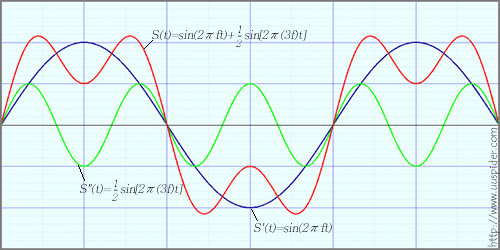## 傅里叶变换的提出

Fig.1## 傅里叶变换

$$f(t)$$是$$t$$的周期函数，如果$$t$$满足一定的条件(狄里赫来条件)，则有：

$$F(\omega )=\cal F \it [f(t)]=\int_{- \infty }^{ \infty } f(t) e^{ -i \omega t}{\rm d}t$$

$$f(t)=\cal F \it ^{-1} [F(\omega )]=\frac{1}{2 \pi } \int_{- \infty }^{ \infty } F(\omega ) e^{ i \omega t}{\rm d} \omega$$

$$F(\omega )$$叫做$$f(t)$$的像函数，$$f(t)$$叫做$$F(\omega )$$的像原函数，$$F(\omega )$$是$$f(t)$$的像，$$f(t)$$是$$F(\omega )$$的原像。